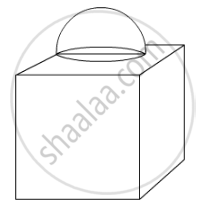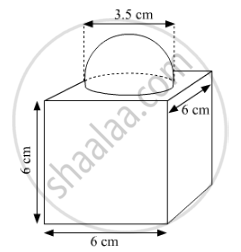# In Fig. 5, is a decorative block, made up two solids – a cube and a hemisphere. The base of the block is a cube of side 6 cm and the hemisphere fixed on the top has diameter of 3.5 cm. Find the total surface area of the bock - Mathematics

In Fig. 5, is a decorative block, made up two solids – a cube and a hemisphere. The base of the block is a cube of side 6 cm and the hemisphere fixed on the top has diameter of 3.5 cm. Find the total surface area of the bock (Use pi=22/7)#### SolutionSurface area of the block = Total surface area of the cube − Base area of the hemisphere + Curved surface area of the hemisphere

=6×(Edge)2πr2+2πr2

=(63+πr2)

=(216+22/7xx3.5/2xx3.5/2)

=(216+9.625)

=225.625 cm2

Concept: Surface Area of a Combination of Solids
Is there an error in this question or solution?
2015-2016 (March) Delhi Set 1

Share